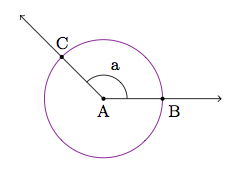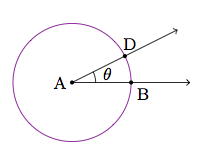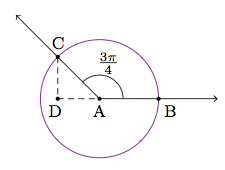# Trigonometric functions for arbitrary angles (radians)

Alignments to Content Standards: F-TF.A.2

Below is a picture of a right triangle with $a$ the measure of angle $A$:Joyce knows that the sine of $a$ is the length of the side opposite $A$ divided by length of the hypotenuse : $$\sin{a} = \frac{|BC|}{|AC|}.$$

Joyce says, ''the sine of an obtuse angle does not make any sense because I can't make a right triangle with an obtuse angle.''

1. Draw a picture and explain how Joyce might define the sine of an obtuse angle.
2. What are $\sin{\frac{3\pi}{4}}$ and $\sin{\pi}$? Why?

## IM Commentary

The purpose of this task is to examine trigonometric functions for obtuse angles. The values $\sin{x}$ and $\cos{x}$ are defined for acute angles by referring to a right triangle one of whose acute angles measures $x$. For an obtuse angle, no such triangle exists and so an alternate definition is required.

Prior to working on this task, students should have experience working with trigonometric functions and how they relate to the unit circle. The task provides a means to assess student learning of these definitions. (Another task using degrees, F-TF Trigonometric functions for arbitrary angles (degrees), can be used to introduce the idea.) One advantage to working with points on the unit circle is that sine and cosine of angles like 0 and $\pi$ still make sense even though they do not belong to any triangle.

## Solution

1. Joyce is right that there is no right triangle with an angle of measure $a$ if $a \gt 90$. She is also right that that the definition of $\sin{a}$ in terms of right triangles does not make sense. Instead, we can begin by drawing an angle of measure $a$, inscribed in a circle of radius $1$, with ray $\overrightarrow{AB}$ along the positive $x$-axis:Consider an acute angle $BAD$ of measure $\theta$, inscribed in a circle,The coordinates of $D$ are ($\cos{\theta}$, $\sin{\theta}$). We can use this fact to make sense of $\sin{a}$ and $\cos{a}$ for the obtuse angle $a$. In other words, for the obtuse angle $a$ we define $\sin{a}$ and $\cos{a}$ so that $$C = (\cos{a}, \sin{a}).$$ Looking at the picture, we can see that for angles with measure $90 \lt a \lt 180$, we will have $\sin{a} \gt 0$ and $\cos{a} \lt 0$.

2. The circumference of the unit circle is $2\pi$ so an angle of $\pi/2$ is a right angle and $\pi/4$ is half of a right angle or 45 degrees. Below is a picture of an angle of measure $3\pi/4$:If the purple circle is a unit circle, then from part (a) we have $\sin{3\pi/4} = |CD|$ and $\cos{3\pi/4} = -|AD|$. We have that $\triangle ADC$ is a 45-45-90 triangle and so $C = (-\sqrt{2}/2,\sqrt{2}/2)$. So we have $\sin{3\pi/4} = \sqrt{2}/2$ and $\cos{3\pi/4} = -\sqrt{2}/{2}$.

The circumference of the unit circle is $2\pi$ so an angle of $\pi$ is half of the circle or 180 degrees. An angle of measure $\pi$ is picured below and it is a line:The coordinates of $C$ are (-1,0) so $\sin{\pi} = 0$ and $\cos{\pi} = -1$.